# 【VRP】基于matlab禁忌搜索算法求解初始点和终点确定的取送货路径问题【含Matlab源码 1224期】

## 一、禁忌搜索算法简介

1 引言 一个问题的求解过程就是搜索，它是人工智能的一个基本问题，而人工智能在各应用领域中被广泛地使用。现在搜索技术渗透在各种人工智能系统中，可以说没有哪一种人工智能的应用不用搜索方法。 禁忌搜索算法(Tabu Search or Taboo Search， TS) 的思想最早由美国工程院院士Glover教授于1986年提出[] ， 并在1989年和1990年对该方法做出了进一步的定义和发展[2-4]。在自然计算的研究领域 中，禁忌搜索算法以其灵活的存储结构和相应的禁忌准则来避免迂回搜索，在智能算法中独树一帜，成为一个研究热点，受到了国内外学者的广泛关注。迄今为止，禁忌搜索算法在组合优化、生产调度、机器学习、电路设计和神经网络等领域取得了很大的成功，近年来又在函数全局优化方面得到较多的研究，并有迅速发展的趋势[5-8]. 所谓禁忌，就是禁止重复前面的操作。为了改进局部邻域搜索容易陷入局部最优点的不足，禁忌搜索算法引入一个禁忌表，记录下已经搜索过的局部最优点，在下一次搜索中，对禁忌表中的信息不再搜索或有选择地搜索，以此来跳出局部最优点，从而最终实现全局优化。禁忌搜索算法是对局部邻域搜索的一种扩展，是一种全局邻域搜索、逐步寻优的算法。 禁忌搜索算法是一种迭代搜索算法，它区别于其他现代启发式算法的显著特点，是利用记忆来引导算法的搜索过程；它是对人类智力过程的一种模拟，是人工智能的一种体现。禁忌搜索算法涉及邻域、 禁忌表、禁忌长度、候选解、藐视准则等概念，在邻域搜索的基础上，通过禁忌准则来避免重复搜索，并通过藐视准则来赦免一些被禁忌的优良状态，进而保证多样化的有效搜索来最终实现全局优化。

2禁忌搜索算法理论 2.1局部邻域搜索 局部邻域搜索是基于贪婪准则持续地在当前的邻域中进行搜索，虽然其算法通用，易于实现，且容易理解，但其搜索性能完全依赖于邻域结构和初始解，尤其容易陷入局部极小值而无法保证全局优化。 局部搜索的算法可以描述为：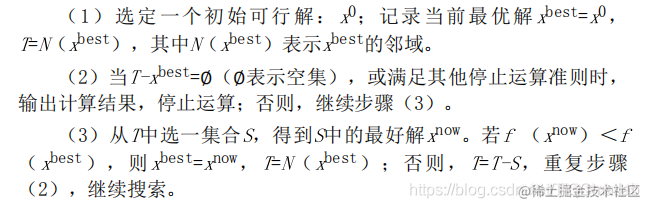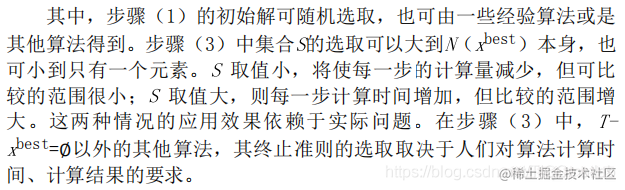这种邻域搜索方法易于理解，易于实现，而且具有很好的通用性，但是搜索结果的好坏完全依赖于初始解和邻域的结构。若邻域结构设置不当，或初始解选择不合适，则搜索结果会很差，可能只会搜 索到局部最优解，即算法在搜索过程中容易陷入局部极小值。因此，若不在搜索策略上进行改进，要实现全局优化，局部邻域搜索算法采用的邻域函数就必须是“完全”的，即邻域函数将导致解的完全枚 举。而这在大多数情况下是无法实现的，而且穷举的方法对于大规模问题在搜索时间上也是不允许的。为了实现全局搜索，禁忌搜索采用允许接受劣质解的策略来避免局部最优解。 2.2禁忌搜索 禁忌搜索算法是模拟人的思维的一种智能搜索算法，即人们对已搜索的地方不会再立即去搜索，而是去对其他地方进行搜索，若没有找到，可再搜索已去过的地方。禁忌搜索算法从一个初始可行解出 发，选择一系列的特定搜索方向(或称为“移动”)作为试探，选择使目标函数值减小最多的移动。为了避免陷入局部最优解，禁忌搜索中采用了一种灵活的“记忆”技术，即对已经进行的优化过程进行记录，指导下一步的搜索方向，这就是禁忌表的建立。禁忌表中保存了最近若干次迭代过程中所实现的移动，凡是处于禁忌表中的移动，在当前迭代过程中是禁忌进行的，这样可以避免算法重新访问在最近若 干次迭代过程中已经访问过的解，从而防止了循环，帮助算法摆脱局部最优解。另外，为了尽可能不错过产生最优解的“移动”，禁忌搜索还采用“特赦准则”的策略。 对一个初始解，在一种邻域范围内对其进行一系列变化，从而得到许多候选解。从这些候选解中选出最优候选解，将候选解对应的目标值与“best so far”状态进行比较。若其目标值优于“best sofar”状态， 就将该候选解解禁， 用来替代当前最优解及其“best sofar”状态， 然后将其加入禁忌表， 再将禁忌表中相应对象的禁忌长度改变：如果所有的候选解中所对应的目标值都不存在优于“best sofar”状态， 就从这些候选解中选出不属于禁忌对象的最佳状态， 并将其作为新的当前解，不用与当前最优解进行比较，直接将其所对应的对象作为禁忌对象，并将禁忌表中相应对象的禁忌长度进行修改。 2.3禁忌搜索算法的特点 禁忌搜索算法是在邻域搜索的基础上，通过设置禁忌表来禁忌一些已经进行过的操作，并利用藐视准则来奖励一些优良状态，其中邻域结构、候选解、禁忌长度、禁忌对象、藐视准则、终止准则等是影 响禁忌搜索算法性能的关键。邻域函数沿用局部邻域搜索的思想，用于实现邻域搜索；禁忌表和禁忌对象的设置，体现了算法避免迂回搜索的特点：藐视准则，则是对优良状态的奖励，它是对禁忌策略的一种放松。 与传统的优化算法相比，禁忌搜索算法的主要特点是： (1)禁忌搜索算法的新解不是在当前解的邻域中随机产生，它要么是优于“best so far”的解， 要么是非禁忌的最佳解， 因此选取优良解的概率远远大于其他劣质解的概率。 (2)由于禁忌搜索算法具有灵活的记忆功能和藐视准则，并且在搜索过程中可以接受劣质解，所以具有较强的“爬山”能力，搜索时能够跳出局部最优解，转向解空间的其他区域，从而增大获得更好的全局最优解的概率。因此，禁忌搜索算法是一种局部搜索能力很强的全局迭代寻优算法。

2.4禁忌搜索算法的改进方向 禁忌搜索是著名的启发式搜索算法，但是禁忌搜索也有明显的不足，即在以下方面需要改进： (1)对初始解有较强的依赖性，好的初始解可使禁忌搜索算法在解空间中搜索到好的解，而较差的初始解则会降低禁忌搜索的收敛速度。因此可以与遗传算法、模拟退火算法等优化算法结合，先产生较好的初始解，再用禁忌搜索算法进行搜索优化。 (2)迭代搜索过程是串行的，仅是单一状态的移动，而非并行搜索。为了进一步改善禁忌搜索的性能，一方面可以对禁忌搜索算法本身的操作和参数选取进行改进，对算法的初始化、参数设置等方面实 施并行策略，得到各种不同类型的并行禁忌搜索算法：另一方面则可以与遗传算法、神经网络算法以及基于问题信息的局部搜索相结合。 (3)在集中性与多样性搜索并重的情况下，多样性不足。集中性搜索策略用于加强对当前搜索的优良解的邻域做进一步更为充分的搜索，以期找到全局最优解。多样性搜索策略则用于拓宽搜索区域，尤 其是未知区域，当搜索陷入局部最优时，多样性搜索可改变搜索方向，跳出局部最优，从而实现全局最优。增加多样性策略的简单处理手段是对算法的重新随机初始化，或者根据频率信息对一些已知对象进行惩罚。

3 禁忌搜索算法流程 简单禁忌搜索算法的基本思想是：给定一个当前解(初始解)和一种邻域，然后在当前解的邻域中确定若干候选解；若最佳候选解对应的目标值优于“best so far”状态， 则忽视其禁忌特性， 用它替代当前解和“best so far”状态， 并将相应的对象加入禁忌表， 同时修改禁忌表中各对象的任期：若不存在上述候选解，则在候选解中选择非禁忌的最佳状态为新的当前解，而无视它与当前解的优劣，同时将相应的对象加入禁忌表，并修改禁忌表中各对象的任期。如此重复上述迭代搜索过程，直至满足停止准则。其算法步骤可描述如下： (1)给定禁忌搜索算法参数，随机产生初始解x，置禁忌表为空。 (2)判断算法终止条件是否满足：若是，则结束算法并输出优化结果：否则，继续以下步骤。 (3)利用当前解的邻域函数产生其所有(或若干)邻域解，并从中确定若干候选解。 (4)对候选解判断藐视准则是否满足：若满足，则用满足藐视准则的最佳状态y替代x成为新的当前解，即x=y，并用与y对应的禁忌对象替换最早进入禁忌表的禁忌对象， 同时用y替换“best so far”状态，然后转步骤(6)：否则，继续以下步骤。 (5)判断候选解对应的各对象的禁忌属性，选择候选解集中非禁忌对象对应的最佳状态为新的当前解，同时用与之对应的禁忌对象替换最早进入禁忌表的禁忌对象。 (6)判断算法终止条件是否满足：若是，则结束算法并输出优化结果：否则，转步骤(3)。 禁忌搜索算法的运算流程如图8.1所示。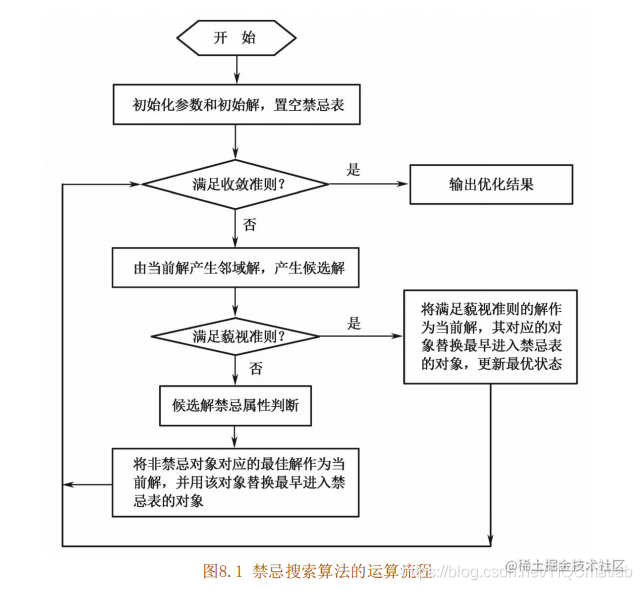4 关键参数说明 一般而言，要设计一个禁忌搜索算法，需要确定算法的以下环节：初始解、适配值函数、邻域结构、禁忌对象、候选解选择、禁忌表、禁忌长度、藐视准则、搜索策略、终止准则[10，11]。面对如此众 多的参数，针对不同邻域的具体问题，很难有一套比较完善的或非常严格的步骤来确定这些参数。 初始解 禁忌搜索算法可以随机给出初始解，也可以事先使用其他启发式算法等给出一个较好的初始解。由于禁忌搜索算法主要是基于邻域搜索的，初始解的好坏对搜索的性能影响很大。尤其是一些带有很复杂 约束的优化问题，如果随机给出的初始解很差，甚至通过多步搜索也很难找到一个可行解，这时应该针对特定的复杂约束，采用启发式方法或其他方法找出一个可行解作为初始解；再用禁忌搜索算法求解，以提高搜索的质量和效率。也可以采用一定的策略来降低禁忌搜索对初始解的敏感性。 适配值函数 禁忌搜索的适配值函数用于对搜索进行评价，进而结合禁忌准则和特赦准则来选取新的当前状态。目标函数值和它的任何变形都可以作为适配值函数。若目标函数的计算比较困难或耗时较长，此时可采 用反映问题目标的某些特征值来作为适配值，进而改善算法的时间性能。选取何种特征值要视具体问题而定，但必须保证特征值的最佳性与目标函数的最优性一致。适配值函数的选择主要考虑提高算法的效率、便于搜索的进行等因素。 邻域结构 所谓邻域结构，是指从一个解(当前解)通过“移动”产生另一个解(新解)的途径，它是保证搜索产生优良解和影响算法搜索速度的重要因素之一。邻域结构的设计通常与问题相关。邻域结构的设计方法很多，对不同的问题应采用不同的设计方法，常用设计方法包括互换、插值、逆序等。不同的“移动”方式将导致邻域解个数及其变化情况的不同，对搜索质量和效率有一定影响。

## 二、部分源代码

``````clear;
CityNum=22;
[dislist,Clist]=tsp(CityNum);
Tlist=zeros(CityNum);%禁忌表(tabu list)
cl=60;%保留前cl个最好候选解
bsf=Inf;
tl=50; %禁忌长度(tabu length)
l1=100;%候选解(candidate),不大于n*(n)/2(全部领域解个数)
S0=randperm(CityNum);
S0(:,1)=0;
S0(:,CityNum)=CityNum-1;
S0(:,2:CityNum-1)=randperm(CityNum-2);
S0=S0+ones;
S=S0(:,2:CityNum-1);
Num=length(S);
BSF=S0;
Si=zeros(l1,Num);
StopL=100; %终止步数
p=1;
clf;
figure(1);
while (p<StopL+1)
if l1>Num*(Num)/2
disp('候选解个数,不大于n*(n)/2(全部领域解个数)！ 系统自动退出！');
l1=(Num*(Num)/2)^.5;
break;
end
ArrS(p)=CalDist(dislist,S);
i=1;
A=zeros(l1,2);
while i<=l1
M=Num*rand(1,2);
M=ceil(M);
if M(1)~=M(2)
m1=max(M(1),M(2));m2=min(M(1),M(2));
A(i,1)=m1;A(i,2)=m2;
if i==1
isdel=0;
else
for j=1:i-1
if A(i,1)==A(j,1)&&A(i,2)==A(j,2)
isdel=1;
break;
else
isdel=0;
end
end
end
if ~isdel
i=i+1;
else
i=i;
end
else
i=i;
end
end

for i=1:l1
Si(i,:)=S;
Si(i,[A(i,1),A(i,2)])=S([A(i,2),A(i,1)]);
CCL(i,1)=i;
CCL(i,2)=CalDist(dislist,Si(i,:));
CCL(i,3)=S(A(i,1));
CCL(i,4)=S(A(i,2));
end
[fs fin]=sort(CCL(:,2));
for i=1:cl
CL(i,:)=CCL(fin(i),:);
end

if CL(1,2)<bsf  %藐视准则(aspiration criterion)
bsf=CL(1,2);
S=Si(CL(1,1),:);
BSF(:,2:CityNum-1)=S;
for m=1:Num
for n=1:Num
if Tlist(m,n)~=0
Tlist(m,n)=Tlist(m,n)-1;
end
end
end
Tlist(CL(1,3),CL(1,4))=tl;
else
for i=1:cl
if Tlist(CL(i,3),CL(i,4))==0
S=Si(CL(i,1),:);
for m=1:Num
for n=1:Num
if Tlist(m,n)~=0
Tlist(m,n)=Tlist(m,n)-1;
end
end
end
Tlist(CL(i,3),CL(i,4))=tl;
break;
end
end
end

Arrbsf(p)=bsf;
drawTSP(Clist,BSF,bsf,p,0);
p=p+1;
end
BestShortcut=BSF;

theMinDistance=bsf
figure(2);
plot(Arrbsf,'r'); hold on;
plot(ArrS,'b');grid;
title('搜索过程');
legend('最优解','当前解');
function F=CalDist(dislist,s)
n=size(s,2);
v1=0.8;%装卸速度
v2=20;%行车速度
w1=0.9;%比重
w2=3;
w3=6;
c=20;%固定损耗成本
r=0.025;%车损与载重质量的损耗系数
q=[0,5,0.5,1,10,4.7,4,1,2,5.5,4,1.5,10,11.1,2,1.4,2.9,0.1,4.7,2,7.9,0];%按照1-30个站点顺序
t1=[0,9.2,10.7,5.9,8.0,9.4,8.8,10.1,11.2,12.0,12.9,13.4,14.2,15.0,16.5,14.3,13.5,13,14.2,16.2,15.3,17];
t2=[34.0,10.0,12.1,6.3,8.5,9.9,9.7,10.9,11.7,12.6,19.4,13.9,14.9,15.5,17.4,18.2,18.9,19.1,19.8,20.4,20.9,23];
q1=zeros(1,n);%更新后的站点顺序
t11=zeros(1,n);
t21=zeros(1,n);
z1=zeros(1,n);%存储超出时间窗的
z2=zeros(1,n);
t=zeros(1,n);%每个站点的到达时间
DistanV=0;%配送成本
loss=0;%车损成本
for i1=1:n
k=find(s(:,:)==i1);
q1(:,k)=q(:,i1);
t11(:,k)=t1(:,i1);
t21(:,k)=t2(:,i1);
end
q1;
t11;
t21;
for j=1:(n-1)
DistanV=DistanV+dislist(s(j),s(j+1));
t(:,j+1)=t(j)+(dislist(s(j),s(j+1))/v2)+max(0,t11(:,j)-t(:,j))+q1(:,j)/v1;
loss=loss+c*(1+r*q1(:,j));
end
DistanV=DistanV+dislist(s(n),s(1));
loss=loss+c;
t(:,1)=t(:,n)+(dislist(s(n),s(1))/v2)+max(0,t11(:,n)-t(:,n))+q1(:,n)/v1;
t;
for m=1:n
if t11(:,m)-t(:,m)>0
z1(:,m)=z1(:,m)+t11(:,m)-t(:,m);
end
if t(:,m)-t21(:,m)>0
z2(:,m)=z2(:,m)+t(:,m)-t21(:,m);
end
end
function [DLn,cityn]=tsp(n)
CityNum=22;
city=[1304,1312;
3488,1535;
3326,1556;
3238,1229;
4196,1004;
4386,570;
3007,1970;
2562,1756;
3918,2179;
4061,2370;
3780,2212;
3676,2578;
4029,2838;
4263,2931;
3429,1908;
3394,2643;
3439,3201;
2935,3240;
3140,3550;
2780,2092;
676,1978;
2449,748];
for i=1:CityNum
for j=1:CityNum
DL(i,j)=((city(i,1)-city(j,1))^2+(city(i,2)-city(j,2))^2)^0.5;
end
end
DLn=DL;
cityn=city;

## 三、运行结果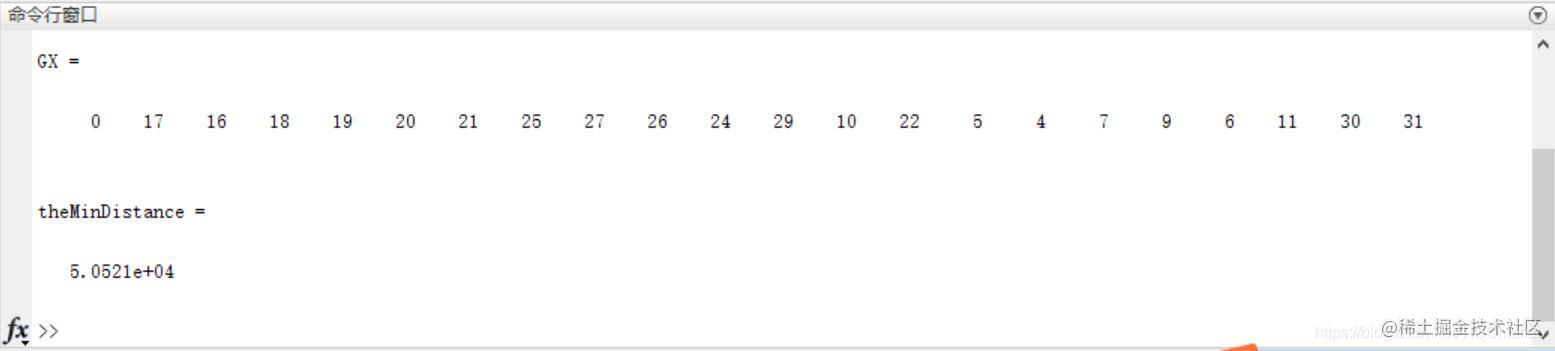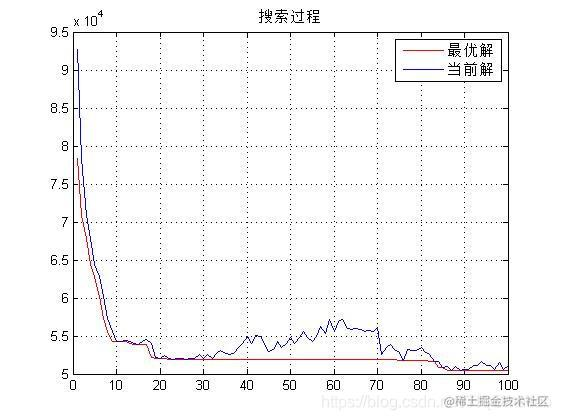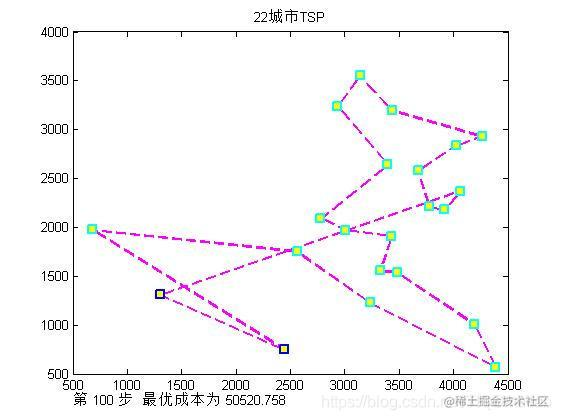## 四、matlab版本及参考文献

1 matlab版本 2014a

2 参考文献  包子阳,余继周,杨杉.智能优化算法及其MATLAB实例（第2版）[M].电子工业出版社，2016. 张岩,吴水根.MATLAB优化算法源代码[M].清华大学出版社，2017.